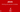# Working with Numeric Variables in javaNumerical values are primitive data type in java. Because its primitive data type so it will also extend these properties:

1. A simple value, only one value will be stored not many.
2. Value will be stored in fastest available memory.
3. Numerical, logical or single character will be stored.
4. Most of the numeric values are stored as primitive data type.
5. Primitive data type is also written in lowercase.

## Below are the types of numeric Variables in java:

• int (4 bytes)
• long (8 bytes)
• float (4 bytes)
• double (8 bytes)
• byte (1 byte)
• short (2 bytes)

## How to set values to these data types?

• int a = 50;
• byte b = 2;
• short s = 20;
• long l = 200L;
• float f = 100.9f;
• double d = 100.9d;

## Helper classes in java

Helper classes are those who help data types. Java class library include numeric helper classes. Helper classes basically includes conversation and output tools. If we want to convert data type then we can use helper classes.

## Below is table for particular helper classes for all data types.

 Data Type Helper Class byte Byte short Short int Integer long Long float Float double Double
Double helper class provide tools to convert float value to double value. As shown below:

## How to use numeric helper class?

• double d = 123.4d;
• Double dbl = new Double(d);
• byte b = dbl.byteValue();
• int i = dbl.intValue();
• float f = dbl.floatValue();
• String s = dbl.toString();

## What are the default value of numeric values if we don’t initialize variable?

The default value of any numeric data type is 0. Ok let’s demonstrate that default value of any numeric data type is 0.

Here is example:

class NumericTest{
public static void main(String[] args){
int a;
System.out.println("value of numeric is : "+a);
}
}

and save this file as “NumericTest.java”, now compile this and check output

And you can see, in output value is 0. (or you will get compile time error that variable not initialized)